MORE SITES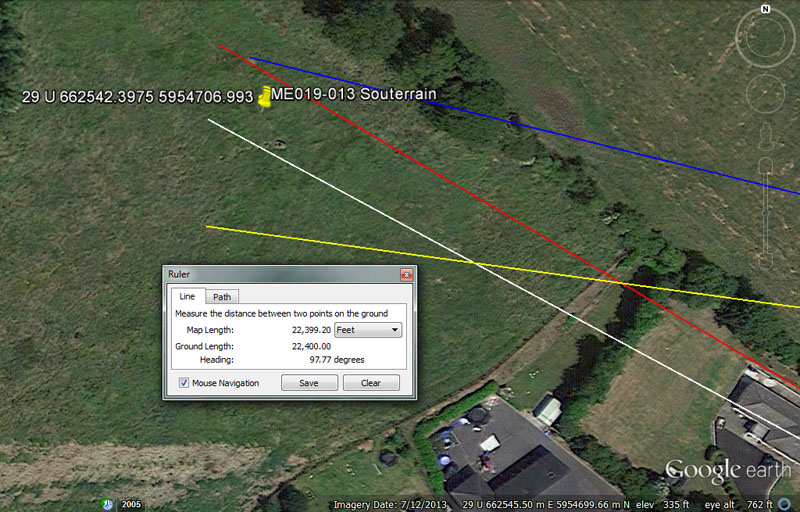This field at Slane offered welcome souterrain accommodation for wet & weary, wayfaring students at the end of a long day of finding their elusive objectives.

From Knowth = 11200’ @ 118.125-degrees.

This distance is 1 & 2/3rds Irish miles. It is also 1/11664th of the equatorial circumference of the Earth (Note: the Roman foot was 11.664"). At a very late era 112 lbs became the hundredweight (cwt) or 1/20th of the 2240 lb ton weight. Most of the standards adopted at late eras in Britain were based upon former old standards, handed down from remote antiquity.

The degree angle is lunar and 118.125-days would be 4 lunar months. Interestingly, the Khafre Pyramid's architectural design was founded upon this length increment. The pyramid incorporated the 3,4,5 triangle principle used by tradesmen from time immemorial, where half the base length was 354.375' (118.125' X 3); from the centre of the pyramid vertically to the apex was 472.5' (118.125' X 4) and the slope diagonal distance from the Apex to the base was 590.625' (118.125' X 5).

From Newgrange = 15360’ @ 120 – 300-degrees.

A distance of 15360' would equate to 1/8505th of the 24883.2-mile (of 5250') circumference, whereas 153.6-miles would be 1/162nd of the circumference.

The outwards and returning degree angles are 1/3rd & 5/6ths segmentations, respectively, of the 360-degree compass.

From Dowth Mound = 19200’ @ 103.125-degrees.

A mathematical progression based upon 12, 24, 48, etc., will generate the 192 or 1536 values and 19200' equates to 1/6804th of the 24883.2-mile equatorial circumference. The values 850.5 & 6804 are, of course, related to the 6804-day lunar nutation cycle. The sum of 192' would be 1/35th of the ancient Irish mile of 6720'.

The angle of 103.125-degrees is in homage to the 20.625" Egyptian Royal cubit (10.3125" X 2).

From Dowth Henge = 22400’ @ 277.77777-degrees (97.7777 return).

The distance to this field souterrain from Dowth Henge is exactly twice the distance as from Knowth chambered mound. In one minute of equatorial arc (6048') the sum of 224' would be 1/27th part.

The encoding within the angle of 277.7777-degrees (277 & 7/9ths) is navigational and this value would be 1/9th of 2500. The return angle of 97.7777-degrees 97 & 7/9ths) would be 1/9th of 880. These kinds of number manipulations and exercises would have been very important to navigators at sea, who would need to be engaged in constant mental arithmetic when working out boat speed and distance traveled within a sea leg.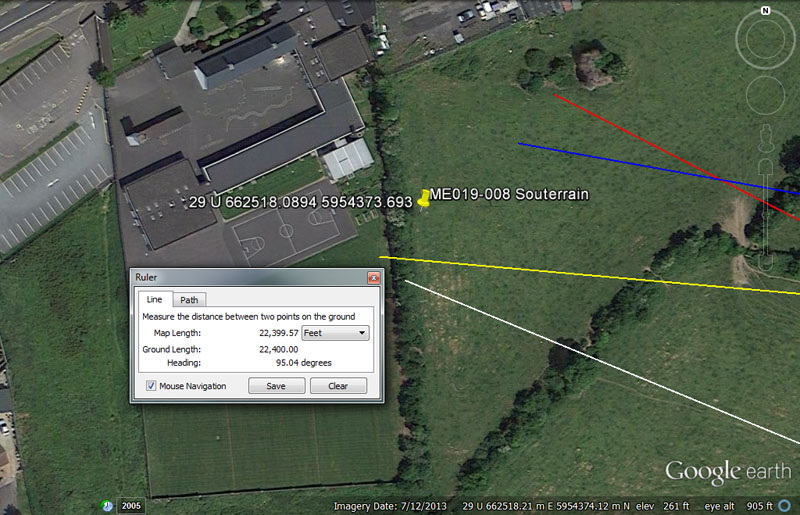Yet another souterrain complex lay in this Slane field and the orienteers, venturing out from the main centres, could have located it on the following coded vectors.

From Knowth = 10800’ @ 112.5-degrees.

The distance of 10800' would equate to 1/12096th of the 24883.2-mile (of 5250') equatorial circumference of the world. The value 12096 is twice 6048' (1-minute of arc), which means that half the equatorial circumference is 10800 X 6048'. There are 21600 minutes of arc in the full circumference (10800 X 2).

The degree angle is self explanatory as 5/16ths of a 360-degree circle.

From Newgrange = 14850’ @ 116.64 or 116.6666-degrees.

This distance is half an ancient Scottish mile of 5940'.

The degree angle could encode the so-called Roman foot of 11.664" or, alternatively, 1/3 of 350.

From Dowth Mound = 19008’ @ 100 - 280-degrees.

Again, the value 19008' (3 X 6336') relates to measuring the equatorial circumference, under 3 different readings or navigational methods, in Egyptian Royal Cubits. Likewise, when voyaging at sea in legs of 1-minute of arc, then converting the completed leg to a circumference for positional plotting, we have 6048' X 22/7ths (PI) = 19008' or 52.8' per degree of arc.

From Dowth Henge = 22400' distance @ 95.04-degrees.

Again, another repeat of the 22400', which equates to 3 & 1/3rd ancient Irish miles.

The degree angle coding is mult-use, but one example would be that 95040' equates to 18 miles of 5280' each.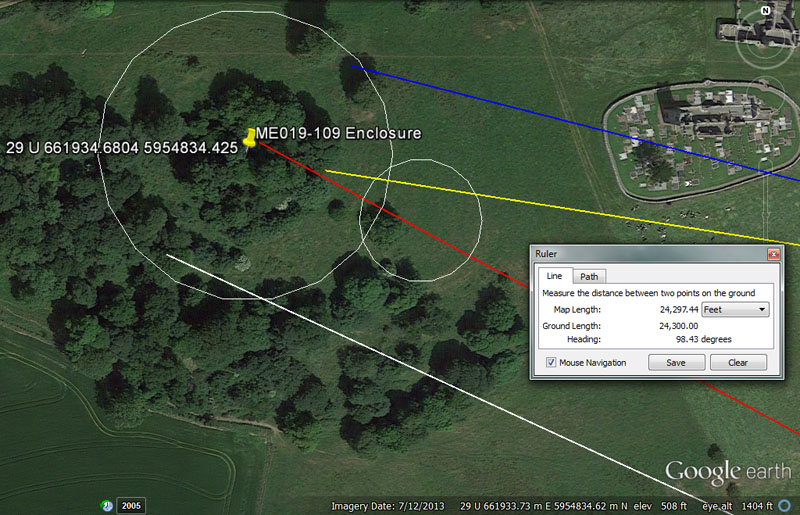At the centre of the giant enclosure atop Slane Hill is the burial mound of the Fir Bolg king Slaine, who was killed upon the hill and is believed to have been buried there. His burial mound was named Dumbach Slaine (or the burial mound of Slaine in literature) and probably resides under the present, higher mound, increased in size as a defensive motte and sentinel position by Richard de Flemming after the Norman invasion.

From the, possible, burial mound of Slaine, king of the Fir Bolg's, the distance to the crown of the Hill of Tara is exactly 10-miles (of 5250' each) at a coded return angle of 15.84-degrees.

The degree angle is navigational and 15840' would be 3-miles of 5280'.

From Knowth = 13200’ @ 295.3125-degrees.

This distance is also navigational and 13200' is 2.5-miles of 5280'.

The degree angle is, of course, lunar coding and 29.53125-days (29 & 17/32nds) is the duration of a lunar month to an accuracy of 1-minute in time.

From Newgrange = 17280’ @ 118.125-degrees … small enclosure = 17010’ @ 118.125-degrees.

The value 1728 was very important to ancient calculations and a cubic foot is 1728 cubic inches (12 X 12 X 12). A navigational tutorial related to turning linear distances (legs of travel) into circumferences divisible by 360-degrees was based upon PI @ 1728/550ths. The appears to have been taught at both Silbury Hill, Southern England and Montfortescue in County Meath Ireland in consideration of marked diameters and circumferences. The sum of 172.8-miles would be 1/144th of the 24883.2-mile equatorial circumference. The sum of 1728-years would be 1/15th of the 25920-year Precession of the Equinoxes, etc.

The same vector passes through the smaller enclosure @ 17010'. The sum of 1701-days would be 1/4th of the 6804-day lunar nutation cycle.

The degree angle is also lunar and the sum of 118.125-days would equate to 4-lunar months.

From Dowth Mound = 21120’ @ 283.5-degrees.

The distance is 4-miles of 5280' and the line seems to resolve at or near a marked structure on the hill.

The line from Dowth mound, running on an outwards angle of 283.5-degrees (strong lunar coding), significantly crosses directly over the grave of St. Erc, said to have formerly been a Druid who was converted to Culdee* (later Church of Ireland) Christianity by St. Patrick and appointed as the first Bishop of Slane. St. Erc is believed to have trained St. Brendon the navigator, who sailed to North America and who later became one of the 12 Apostles of Ireland.

*Note: St Patrick was not an emissary from the Roman Christian Church, which did not become dominant in Ireland until 1172 AD.

Saint Erc's grave sits just twenty feet in excess of the highly coded position of 20625' @ 283.5-degrees from the centre of Dowth chambered mound. Two surviving standing stones from a former circle are nearby.

This distance is 1.25 leagues of 16500' (3.125-miles of 5280'). Alternatively, there would be 1.25 intervals of 283.5-days in a lunar year of 354.375-days. Perhaps the coded positioning of St. Erc's grave honoured his true Druidic roots and heritage.

From Dowth Henge = 24300’ @ 98.4375-degrees.

A distance of 24300' would be 5 so-called Roman miles.

The degree angle is lunar coding and 9.84375-days (9 & 27/32nds) would be 1/3rd of a lunar month. Likewise, 196.875-grains (196 & 7/8ths) became the weight used by ancient assayers in Egypt, Greece & Rome for their Beqa gold standard. Also 196875' would be 37.5-miles of 5250'.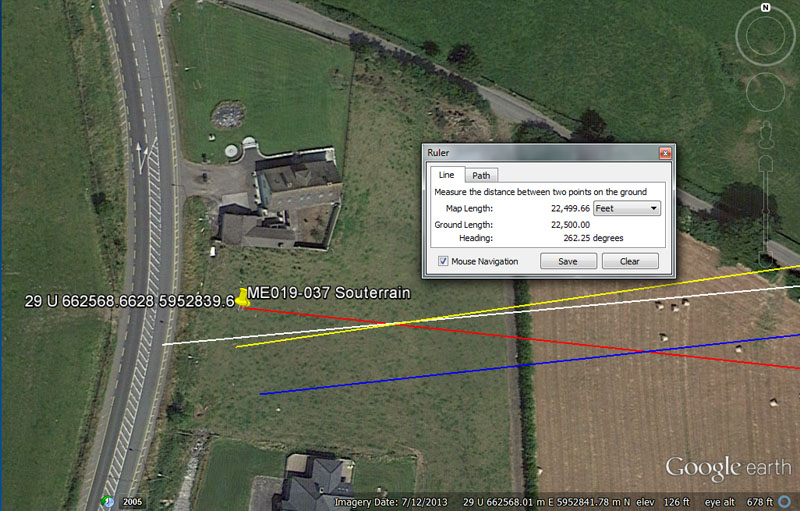There was yet another souterrain hostel awaiting transient students visiting Fennor, on the Southern side of the Boyne from Slane Hill.

From Knowth = 10080’ @ 85.05-degrees.

This distance is 1 & 2/3rds minutes of equatorial arc.

The degree angle is lunar and 850.5-days would be 1/8th of the 6804 lunar nutation cycle.

From Newgrange = 13440' to 13500’ @ 276.48-degrees.

This distance is 2 Irish miles, but tutorials related to 13500' (as shown) could also have been included. The sum of 135-degrees would be 3/8ths of a 360-degree circle.

The degree angle is navigational coding and 276.48-miles would be 1/90th of the 24883.2-mile circumference.

From Dowth Mound = 18900’ @ 84.375-degrees.

This distance is 3 & 1/8th minutes of equatorial arc or 3.6-miles of 5250'. It is also 2 & 13/16th Irish miles, etc.

The value 84.375 (84 & 3/8ths) was much used in lunar calculations and there would be 4.2 intervals of 84.375-days (2024-hours) in a lunar year of 354.375-days. A mathematical progression based upon this value will provide many useful numbers used in lunar calculations.

From Dowth Henge = 22500’ @ 262.5-degrees.

The sum of 2250-miles would be 1/11th of the 24750-mile circumference and 22.5-degrees would be 1/16th of a 360-degree circle, etc.

The degree angle is also navigational and 2625' is half a 5250'-mile.

It was only by thoroughly immersing themselves in these scientific values that initiate navigators could learn the art. It had to be done by-the-numbers.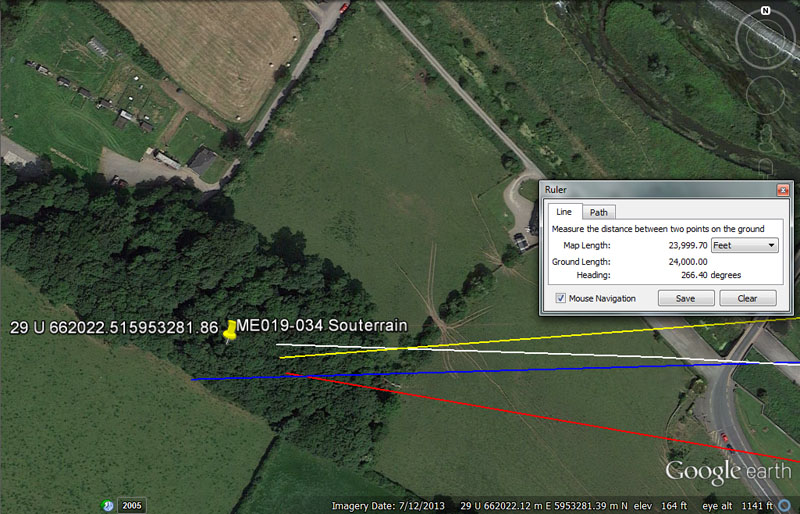And again, under this tree canopy is more souterrain accommodation for orienteers assigned to find the several structures in the vicinity of Fennor

From Knowth = 11664' @ 93.312-degrees.

This distance is 2.4 so-called Roman miles. The Egyptian Theban volume was 11664 cubic inches, whereas their Besha was 1166.4 cu" and Honnu 116.64 cu".

The degree angle is similar coding and the sum of 93312' would be 19.2 Roman miles. The degree angle also encodes a much used value of antiquity that found its way into the weights and measures standards of several civilisations. For example, the Sumerian / Babylonian heavy Talent was 933120-grains. The sum of 933120' would be 1/140th of the 24883.2-mile (130636800') equatorial circumference of the Earth.

From Newgrange = 15360’ @ 101.25-degrees.

A distance of 15360' would equate to 1/8505th of the 24883.2-mile (of 5250') circumference, whereas 153.6-miles would be 1/162nd of the circumference.

There would be 3.5 intervals of 101.25-days in the lunar year of 354.375-days.

From Dowth Mound = 20625’ @ 89.1-degrees.

This distance is 1.25 leagues of 16500' (3.125-miles of 5280').

The 891 value is navigational coding and 8910' would be 1.5 ancient Scottish miles.

From Dowth Henge = 24000’ @ 86.4-degrees.

The distance coding is quite self explanatory.

The degree angle is multi-use for several kinds of calculations. The sum of 8640-years would be 1/3rd of the 25920-year Precession of the equinoxes cycle and 8640' would be 1/7th of 10-minutes of equatorial arc (60480'). The sun is 864,000-miles wide.

ROSSNAREE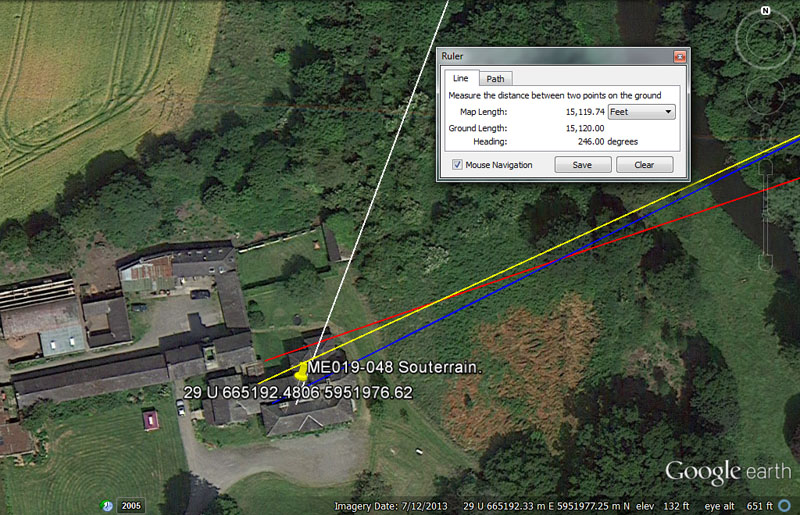Yet another souterrain is to be found beneath this building at Rossnaree.

From Knowth = 4252.5’ @ 20.16-degrees return.

This distance coding is lunar and the sum of 425.25-days would be 1/16th of the 6804-day lunar nutation cycle duration.

The angle coding is navigational and 2016' would be 1/3rd of the 6048' in 1-minute of equatorial arc.

From Newgrange = 5184’ @ 252-degrees.

Again, one of the most used and dynamic numbers of antiquity. The design slope angle of the Great Pyramid was 51.84-degrees. The area that the base of the Great Pyramid covers is 5184 square reeds (of 10.5' each). In the cycle of Precession, 5184-years would be 1/5th of the duration. In navigation at sea, 1-league of travel (16500') would create a circumference of 51840', which is 144' per degree of arc. Also the ancient Greek volume for dry goods called the Hecteus was 518.4 cubic inches, whereas the Jerusalem liquid volume called the Cab was 103.68 cubic inches or 51.84 X 2.

The degree angle is navigational and and 252' was 1/3rd of the length of the Great Pyramid or 1/24th of 1-minute of equatorial arc. It was also 2.5 seconds of arc @ 100.8' each. The sum of 25.2" would be 2 Greek feet or 1 Assyrian cubit. In the 6804-day lunar nutation cycle the sum of 252-days would be 1/27th part.

From Dowth Mound = 11458.33333’ @ 244.4444-degrees.

This value is navigational and in the 24750-mile equatorial circumference the sum of 114.58333 X 6 X 6 X 6 = 24750. Likewise, 1-degree of arc under this navigation method (68.75-miles) ÷ 6 = 11.458333-miles (11 & 11/24ths).

The sum of 244.4444' (244 & 4/9ths) would be 1/9th of 2200'. Initiate students had to know how to manipulate whole numbers and render them down into much reduced fraction form. It all appears to be the "times-table-from-hell", learnt by rote and repetition, but as Julius Caesar attested in regards to the late-era Druidic schools of his day, the students were learning their lessons with "severe exactitude".

Adept users of the varied, manual abacus devices of antiquity could do difficult calculations at about the same speed as it takes for modern students to push the buttons on their electronic calculators.

From Dowth Henge = 15120’ @ 66-degrees.

This distance is, again, 2.5-minutes of equatorial arc or 1/20th the distance between Dowth Henge and Slieve Gullion Mountain's summit trig.

The angle of 66-degrees is in homage to the chain of 66' or furlong of 660', etc. There would be 5 & 5/11ths X 66-degrees in a 360-degree circle.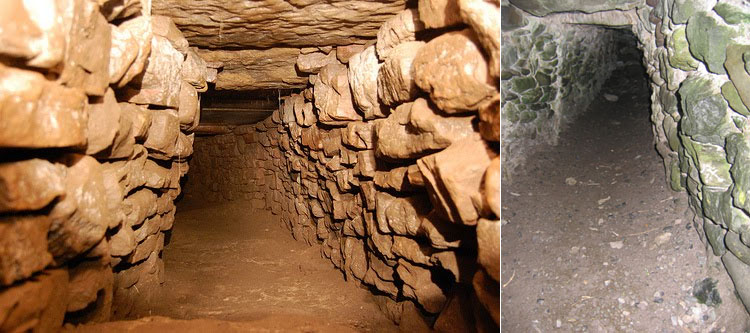It might be added that these souterrain structures were very laboriously built, often incorporating huge wall and overhead lintel stone slabs. Many of the giant stones used in their construction individually weighed several tons and souterrains were definitely not built by ancient farming families for winter storage of crops or the like, but by large teams of burley men.
Souterrain construction entailed excavating huge cubic yardages of soil and rock in order to create reinforced underground passages and rooms, then roofing over the deep trenches and room cavities with heavy wooden oak beams and long stone roofing slabs, before re-covering the tunnel structures with compacted soil.
Temperatures within the souterrains stayed fairly constant, year-round and it's probable these hostels were frequently reprovisioned by the open-air-university support network, so that wayfaring students had ready access to dry food, firewood and water supplies.

Continue